# Meta-Learning (Learn how to Learn)

People learn continuously. We recall relevant skills and adjust them accordingly in handling new tasks. Currently, supervised learning has limited perspective and scope that sound like the “Blind Men and the Elephant Story” — each person’s experience is limited to where he/she touches. For instance, supervised models are often trained to specialize in a specific task and dataset only. To form a better perspective, we should learn how to learn (meta-learning). Reproducing the learning efficiency of humans is one of the holy grail in AI.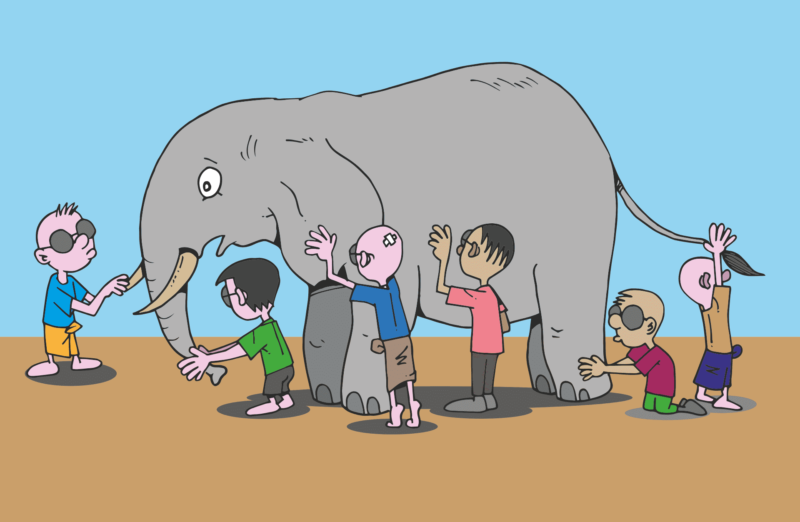Source

Specifically, one big challenge in deep learning (DL) is how can we learn from trained tasks to form transferable knowledge. One obvious solution is to train a model with a meta-training dataset. This dataset contains multiple datasets that correspond to independent tasks from similar problem domains. This helps us to learn the common skill behind them.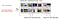Image source

For example, in robotics, we don’t want to train a robotic arm just to hold a plate. Instead, we want independent tasks to learn basic mechanics to hold and move objects. For instance, one training task may be hanging cloth and another to be washing dishes. So, when it deals with an unforeseen object, it can adapt quickly. Instead of calling this process “training”, as in supervised training, it is called meta-training. However, not to raise unrealistic expectations, current state-of-the-art technology trains tasks that are much similar than what we aim for.

In meta-learning, we don’t combine samples from all tasks and perform supervised training. One major differentiator is the learning efficiency for new tasks as humans require far fewer samples to learn new things. In particular, for safety reasons, we need to handle rare events correctly even we have no or only a few new samples to learn from. In general, this requirement will extend to all new tasks. Trained models should adapt and extrapolate quickly to new situations and tasks quickly.

Let’s elaborate on it with a classification example. Given a model trained with a meta-training dataset, we want it to classify new object classes quickly. In meta-testing (equivalent to testing in supervised learning), we are provided with a meta-test dataset D. In this example, the images in D belong to classes that the model never saw before. It includes five images on five different labeled classes, i.e. one image per class. These are the only samples that the model can learn from and when two additional images are presented, the model should classify them into these new classes correctly.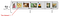Image source (Meta-test dataset D)

# Problem Statement

While this explains our goal well, it is still filled with vague terms. To move forward, let’s see how the problem can be formulated. One possible approach is to extract knowledge/belief from a set of tasks that is transferable in solving other tasks. In machine learning (ML) terms, we want to learn the prior from previous experience. With enough experience, the prior should be rich enough to be refined by a small training set in making accurate posterior (prediction) for new unforeseen tasks.

In the presence of a meta-training dataset Dmeta-train that containing dataset D₁, D₂, … and a meta-test dataset D, our training objective can be written as the MAP (Maximum a posteriori) below.

It finds the best model parameterized by 𝜙* given all the observed samples. For example, if this is a classifier, 𝜙* makes the best predictions against the observed sample labels. But instead of memorizing Dmeta-train, we want to capture its information in another ML model parameterized by θ. The MAP objective of this model will be:

Now, let’s combine both objectives together.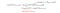Modified from source

Intuitively, we optimize a model parameterized with θ to learn the information encoded in Dmeta-train. Then we adapt from this model to create another model 𝜙 that specialized in a specific task.

The learner θ is called the meta-learner. It discovers the general and transferable principals and 𝜙 is a learner adapted for a specific task.

Let’s elaborate on this strategy with one possible realization. But we will talk about meta-testing first before meta-training.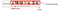Source with Image from Ravi & Larochelle ’17

The meta-testing contains a dataset D specified for a task. It has a support (the training data within a task) and a query (the testing data for a task). Because the term “training” may have multiple meanings in meta-learning, we will use the term support and query as in many meta-learning papers.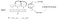Modified from source

In this example, it uses an LSTM to predict 𝜙* from the support. Then 𝜙* will be used as the model parameters for an MLP (a network with fully-connected layers). This MLP, a learner for a specific task, will then make predictions from the query. Intuitively, the LSTM parameterized by θ extracts the task context of D from its support and creates model parameters 𝜙* for the MLP to make predictions. To evaluate a meta-learning algorithm, we can prepare many meta-testing datasets for different tasks to measure its accuracy.

Let’s see how we train the model. Both the LSTM and MLP are trained with a meta-training dataset. This dataset contains many datasets that each dataset Di is responsible for a specific task. In this example, each task contains a classification task for different classes. The support contains n classes with k samples each. This is called n-ways with k-shot. In the example below, it applies 5-ways 1-shot training.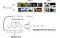Modified from source

For each dataset Di, we use its support as the input to the LSTM and we feed the query into the MLP. We compute the loss from the true labels and the MLP predictions and backpropagate the loss gradient in updating θ.

This can be viewed generally as creating an adapted model parameterized by 𝜙 that is a function of f parameterized by θ* with input form the support of the meta-testing dataset.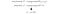Source

This formularization can be understood more intuitively. We have a meta-learner model θ that can be used to create an adapted model 𝜙 from samples of a specific task. Our objective is to learn such a meta-learner that can produce accurate learners with the corresponding task samples.

# Algorithms

Next, we will go through different meta-learning algorithms which are traditionally grouped as

• Black-Box Adaptation/Model-based,
• Optimization-based, and
• Metric-based.

Most Meta-Learning approaches contain an inner loop (step 3) and an outer loop (step 4). The inner loop contains an adaptation objective to adapt the meta-learner model θ to learner model 𝜙 for task i. The outer objective (the meta-objective) is to learn θ so it can be produced a better learner model 𝜙 for a specific task.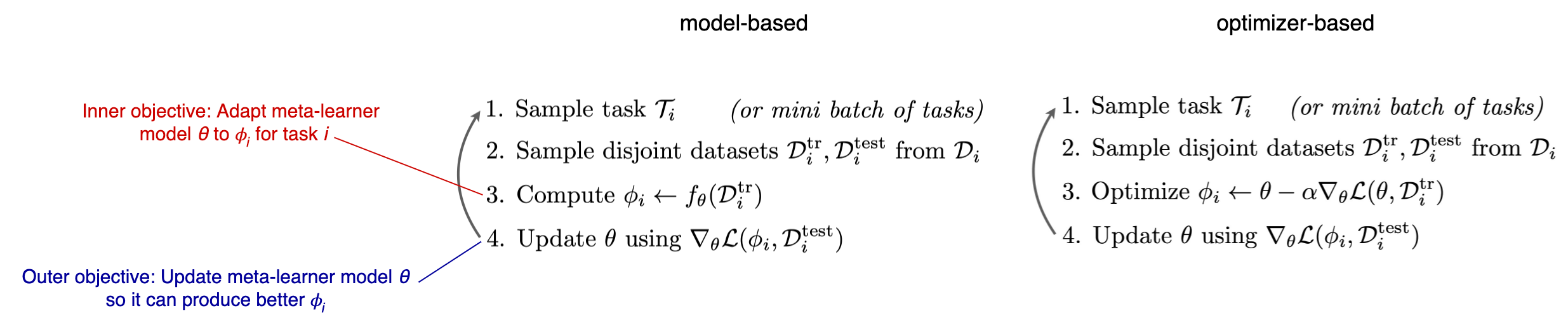Modified from source

# Black-Box Adaptation or Model-Based

Our previous example has described one of the realizations of the model-based approach which generally involves model f and g parameterized by θ and 𝜙respectively. In this approach, f extracts task context, and g predicts the classification.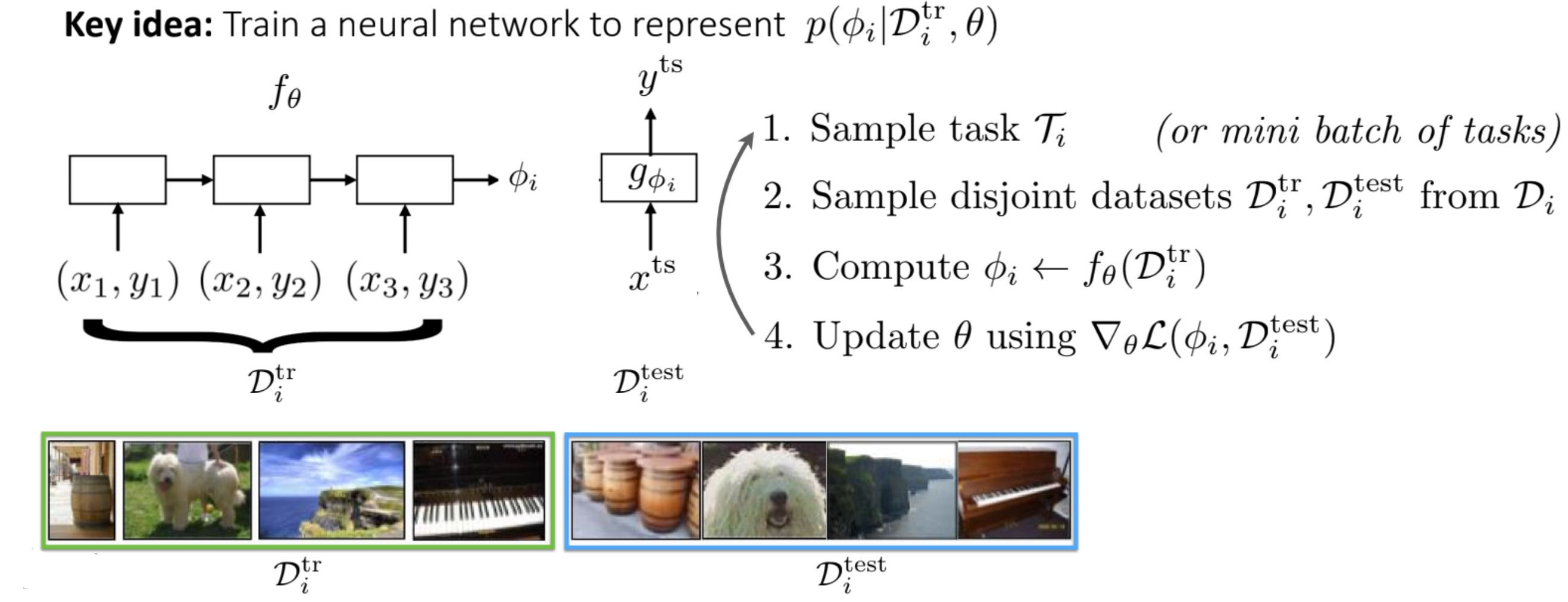Modified from source

In our previous example, f is an LSTM model. But many choices can be used including bi-directional LSTM, Neural Turing Machine (NTM), Self-attention, 1D convolution or simply use a feedforward network and compute the output average of the support.

But some of you may already challenge the scalability of the solution if g is designed to have millions of parameters. For this scenario, we can train f to generate a low-dimensional hᵢ to represent the context of task i and be part of the model parameters for g.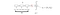Modified from source

So, g is composed of layers that are specialized for a task and layers that are generic to all tasks (the transferable knowledge). As shown below, g is composed of the parameters hᵢ and θg. θg is generally trained during the meta-training with backpropagation and hᵢ is the mentioned task context which is often used as the parameters for the top fully connected layers in g.

Some meta-learning algorithms may be further simplified or approximated. For example, f and g can take on the role of feature extractor and classifier respectively. In other algorithms, f and g can be fused into a single DNN.

We will delay some topics including bi-directional LSTM, self-attention, and 1D temporal convolution for the model-based approach. These design techniques are adapted in many other approaches including optimization-based meta-learning and Bayesian meta-learning. To avoid redundancy, we will describe them with more detailed examples later. But even it is in the context of other approaches, the concept behind them is the same.

Next, we will look into model-based meta-learning using memory.

Neural Turing Machine (NTM)

In computer programming, we use an index to access a memory array. But in AI, we recall memory by contents and similarity. For instance, we assemble a memory object by piecing similar objects in memory together.

NTM uses a controller to extract the feature kt for the input voice xt. This controller can be implemented with an LSTM or a feedforward network. Each row (row ith) in the memory array Mt stores a vector kᵢ to represent a voice. To output (read) a memory object, the controller compares kt with the content of each row (kᵢ). The final memory readout rt will be a weighted sum of rows according to the similarity wᵢ for each row.

Intuitively, this returned object is a normalized collection of objects based on similarity in content. This weight wt(i) uses cosine similarity K[] to measure the similarity of kt with kᵢ and then normalized it with softmax.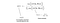Modified from source

where β is another parameter used to amplify/attenuate the similarity measurement. If it is greater than one, it amplifies rows that are more similar. For classification problems, we can use the readout rt as an input to a classifier to predict the object class.

This kind of addressing mode is called content-based addressing. On the other hand, location-based addressing handles traditional variable accesses, like a=b+c. NTM provides many combinations of addressing modes including combining content-based and location-based addressing together. But this is out of our scope in the meta-learning study, please refer to this Neural Turing Machine article and the original paper for more addressing mechanism.

The memory writing in NTM composes of two phases: an erase followed by an add. In erase, we remove part of the memory based on the weight wt(i) and the erase vector et. wt(i) controls which rows and how much to be erased. et controls which components in kᵢ and also how much of them will be erased. The following equation shows how NTM erases part of M from timestep t-1 to timestep t.

The add phase is very similar and uses at to determine which components in kᵢ and how much to be added.

Both et and at follows the concept of the forget gate and input gate in LSTM which uses these gates to control what previous states to forget and what current updates to keep.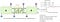Modified from source 1 & 2

In solving DL problems, et and at are trainable parameters output by a DNN, say an MLP network using input from the hidden state ht of the LSTM cell. Since the read and write operations are differentiable in NTM, we can assume many parameters in the paper are trainable using Gradient Descent. But the NTM paper focuses on painting a new architecture design without too many details on the implementation. So we will not get into its implementation details also.

To recap, NTM allows us to store information into memory. But the input space will be too large to feed into the limited memory space. So the controller is trained to produce lower dimension latent factors and cluster similar information together with its write operations. In addition, NTM uses the content-based read to reconstruct objects by similarity even the input has not been seen before.

In another paper called dynamic NTM, it modified this approach to solve the Q&A problem. The controller using GRU to process the facts as continuous vectors. If a query is detected, it uses content-based addressing to read and write information from and to the memory. We just want to demonstrate the possible applications and please refer to the paper for design details.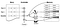Source

Memory-Augmented Neural Networks (MANN)

Let’s integrate the NTM memory network into the meta-learning architecture discussed before.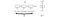Source

In MANN, it encodes the input xᵢ to kᵢ and bound its corresponding memory location with the associated class yᵢ. In testing, it reads the memory for xtest with content-based addressing and outputs the bounded class.

However, this learning process can be easily degraded to supervised learning where the whole model simply learns how to map an input xᵢ to the class yᵢ and the memory is not actually used or trained.

To solve that, MANN delays the supply of the label yᵢ by one timestep.

This forces MANN to learn how to remember information into its memory in which it will bound to its label in the next timestep. Hence, every time when a new class is introduced, MANN can only make a random guess at best. But once it is stored in memory and bounded, MANN can utilize the new information for inference.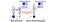Source

In meta-training, MANN will maximize the probability of its final label prediction and backpropagate the loss gradient to train the model.

MANN uses content-based memory addressing for the read and write. But, different from NTM, MANN uses the Least Recently Used Access (LRUA) in writing memory.

• If the information is new, MANN stores it in the least used memory location.
• If the information is an update of the recently acquired information, MANN saves it in the most recently used memory location so it may reuse the space occupied by the older data.

To do that, MANN will utilize the previous read and access patterns in determining whether the information is new or just a modification of the recent activities. Here is the equation

which w is computed from the previous read and write access pattern to compute where to store the information. For simplicity, we will skip the formalization of w, and please refer to the MANN paper for details.

Memorization

The following is the general equation for meta-learning.

As previously discussed, if the datasets are not properly prepared, we can train a network that derives the label from the query directly without the help of the support. In short, we solve the problem with supervised learning and the model θ has already been trained with data similar to the query and it managed to build a function that make predictions from the samples directly.

This contracts our goal of extracting general knowledge in handling unforeseen new tasks with a small sample of support. To avoid such situations, we need to prepare tasks that are mutually exclusive. Information in the support and query alone should not be good enough to make accurate and generalized predictions — a rich prior is needed in the process.

Meta Networks (MetaNet paper)

Now, let’s come back to the general model-based meta-learning again. We will study a learner with parameters partially coming from a meta-learner.

MetaNet can solve both classification and regression problems. But for the ease of explanation, we will focus on the classification problem here. In such a problem, Meta Network composes of an encoder u to extract features and a classifier b to classify these features.

Both u and b contain two sets of weights: fast weights and slow weights. If it is implemented as an MLP, each one will look like: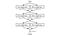Source

If we remove the fast weight layer, it behaves exactly like a regular DNN and in facts, slow weights are trained with the regular gradient descent method with a loss function. These are called slow weights because the gradient descent method is viewed as a slow convergence method.

The fast weights are predicted (inferred) by a meta-learner. The key strategy is to train the meta learner’s DNN d & m to infer the fast weights quickly for a new task. Before generating these weights, it calculates the loss of encoder u and classifier b if only slow weights are used. Then, d & m will use these loss gradients as input respectively to generate the corresponding fast weights.

MetaNet algorithm (Optional)

Below is the detailed algorithm for a single meta-training iteration on a single task. Each task contains a support with N samples and a query with L samples. The notations are a little bit tedious but the algorithm is not too hard to understand but does require some patience. So read them according to your interest level.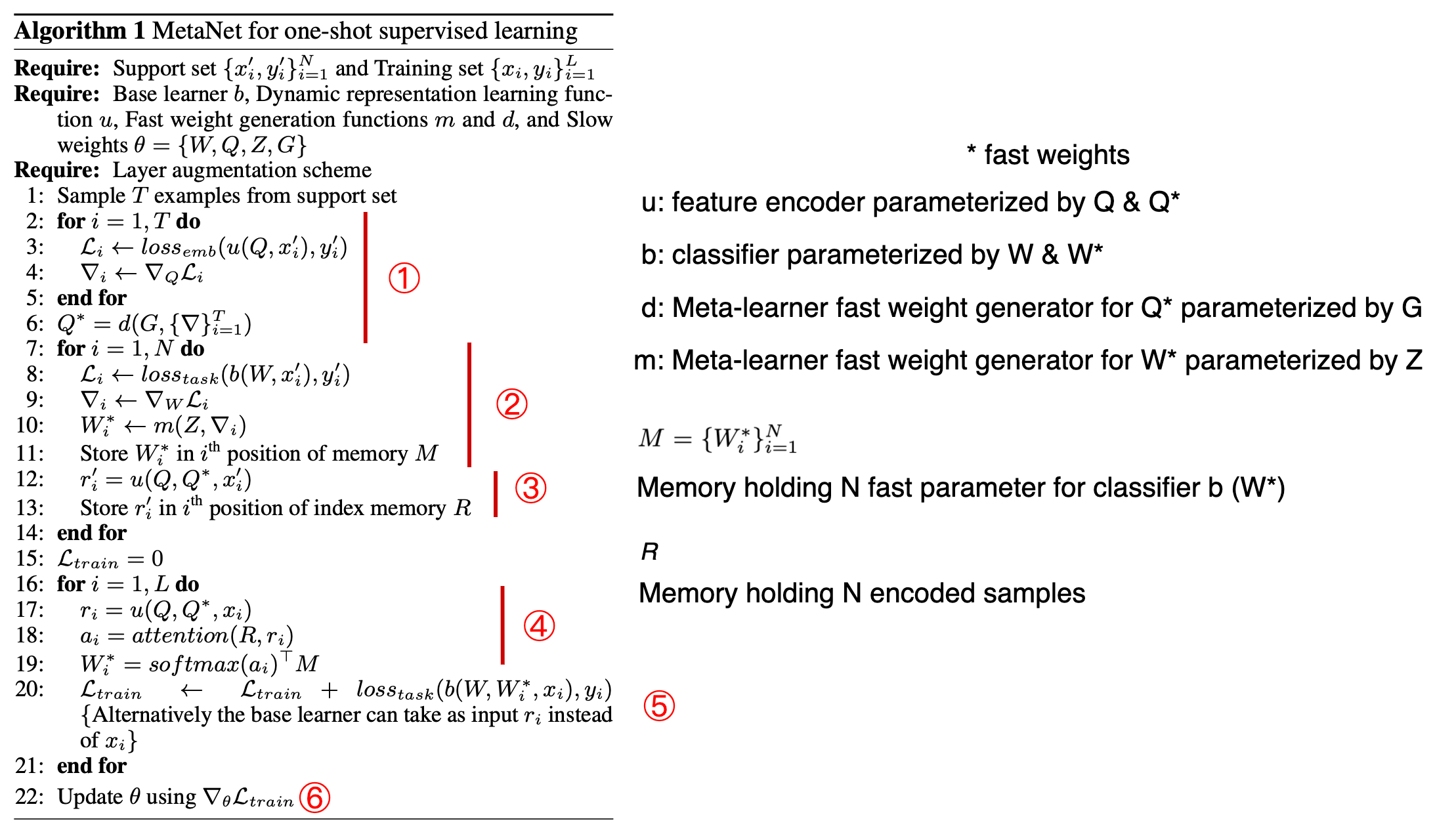Modified from source

MetaNet has a meta-learner composed of two DNNs: DNN d and m is responsible for generating fast weights for the encoder and classifier respectively.

Step ① samples T examples from the support.

Then, MetaNet estimates the loss of its encoder using the slow weights only: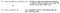Source of the equation

The meta-learner d will use the gradient of this embedding loss as input to infer the encode’s fast weights Q*. This concept allows d to generate fast weights sensitive to a specific task.

Step ② to step ⑤ predicts the fast weight W* for the classifier on each query sample. Intuitively, the fast weights W* for the classifier will be adapted to the current task by finding the the similarities between the support and the specific query. Let’s have an overview before detailing the steps. For each sample i in the support, we compute its encoded feature rᵢ’ and Wᵢ’* (Wᵢ* in the code). Similar to the previous step, Wᵢ’* are predicted by the meta-learner using a loss gradient. But this time, it uses the classifier loss gradient. Then we iterate through each query sample and find its attention (similarities) corresponding to each support readout rᵢ’. Then, this attention will be used to readjust Wᵢ’* to Wᵢ*, i.e. the fast parameters in the classifier will be adapted to the similarities between the support and the specific query.

Here are the fine details. In step ②, we iterate over all N samples in the support.

For each sample, we compute the loss of the classifier using the slow weights W only. The meta-learner m will use the gradient of this loss as input to infer the classifier’s fast weights Wᵢ*. But it creates one gradient and one Wᵢ* per support sample. And the result Wᵢ* will be stored in the ith position of memory M for later use.

In step ③,

we extract the features from the input using the encoder with both fast and slow weights. The result r’ᵢ will be stored in the ith position of memory R. Now R stores the representation r’ᵢ of the support with the corresponding fast weights Wᵢ* for the classifier in memory M.

In step ④,

we iterate over L queries and encode the input as features rᵢ with both fast and slow weights. MetaNet computes the similarity between the query and the support and uses it to readjust the fast weights Wᵢ* for the classifier:

1. MetaNet computes the soft attention focus (similarity) between rᵢ in the query and R which stores the support features.
2. MetaNet uses softmax to convert the result to a probability distribution.
3. MetaNet multiplies the result with M to readjust Wᵢ*.

In short, we estimate W* based on the support and now we adopt these values to the corresponding query based on similarity.

In step ⑤, we compute the loss of the classifier which uses the adjusted fast weights and slow weights in making predictions.

In step ⑥, using the computed loss, we train the slow weights for the encoder and the classifier, as well as the meta learner’s d and m.

Recap

In a memory-based system, the meta-learner collects and encodes our experience into a memory structure. Later, memory is recalled by piecing similar objects in memory together.

In other model-based algorithms, the meta-learner generates

1. weights of a complete model, or
2. the context representing the support of a task.

For the first case above, the weights will become the model parameters of a classifier/regressor DNN. In the second case, the context will be used as weights for a part of the DNN, usually as the last layer. But it either case, the DNNs are trained to make predictions that adapt to a specific task quickly. During meta-testing, we simply repeat the process for an unforeseen task with the hope that the DNNs have already acquired the generic knowledge and can be readapted with a small dataset.

# Meta-Learner Optimizer

Let’s study the second type of Meta-learning approach that focuses on the optimizer. Again, we optimize (train) the meta-learners to adapt to specific tasks easily with minimum data samples.

LSTM-based Meta-Learner Optimizer (paper)

The algorithm below uses an LSTM R for the meta-learner. Some of the concepts are similar to what we discuss in MetaNet. R will predict model parameters for the learner M. But beside the loss gradient, it also uses the loss values, and previous parameters for M as input.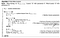Source

In step 7, it selects T samples from the support and makes label predictions for each sample using the learner M. For each sample, it computes its loss ℒ and the corresponding gradient ∇ℒ. The meta-learner R, parameterized by Θ, uses these computed values as input to make a new model parameter proposal for the learner M. But the adopted parameters for M at time t will be a gated combination of the previous parameters and the current proposals (step 10).

We repeat the process for T iterations and uses the last model θT as the learner M. Then, using the query samples, we make predictions with θT and use the loss gradient to update the meta-learner model parameter Θ (step 16).

Model-Agnostic Meta-Learning (MAML)

In Gradient Descent, we use the gradient of the loss or the reward function to update model parameters.

But this learns a particular task rather than finding the fundamental knowledge behind all the tasks. So instead of updating the model immediately, we can wait until a batch of tasks is completed. We later merge all we learned from these tasks for a single update. This approach fulfills the concept of “learn what we learn”.

MAML utilizes this concept to update models. It is simple and it is almost the same as the traditional DL gradient descent with one added line of pseudocode.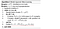Source

MAML collects a batch of weight updates from different tasks and each set of weight updates will propose a new model (step 6). Once this is done, MAML evaluates the loss again for each proposed model with samples from the query (note: the samples come from the query, not the support). MAML sums the loss and computes the gradient w.r.t. θ. Finally, the model parameters θ are updated with gradient descent.

Here is the objective that the meta-leaner is optimized for. It adopts a model for a specific task using the support and then finds θ that can most easily adapt to these tasks according to the query samples.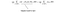Equation source

Conceptually, we train specific learners θᵢ below and we later use their proposal models to train the meta-learner parameterized by θ. The solid line below indicates how the meta-learner converges to its optimal.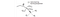Source

Once the training is completed, we can use this meta-model to initialize a learner model in handling new tasks. Then, it can be further finetuned. This finetuning only needs a few examples and a single or a few gradient steps. For example, the finetuning for task 3 will move the optimal point towards the optimal θ₃*. MAML is model agnostic. But for any optimization-based methods to be more effective, the DNN f seems to be narrow and deep (AutoMeta).

SNAIL (A Simple Neural Attentive Meta-Learner paper)

SNAIL meta-learners deploy temporal convolutions (TC) to aggregate information from past experiences and use soft attention to learn which part of the current data should be focused on.

SNAIL models are composed of layers of TC and causal attention. Causality is applied such that current states or actions depend on past histories but not the future. If it is applied to supervised learning, SNAIL takes in a sequence of input and labels (except for the last entry) to predict the label for xt. If the input is an image, a CNN network will be applied to extract the features first.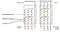Modified from source

A temporal convolution (TC) layer composes of many dense blocks as used in the DenseNet. In DenseNet, the input of a block comes from all previous layers — not just the last layer.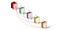Source

Here is the code in concatenating all these Dense blocks together as input to the next layer.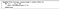Source

A dense block applies a causal 1D-convolution with dilation rate R and D filters. For the next TC layer, the dilation R will be doubled. This expands the receptive field temporally.

Below is how we construct a Dense Block. It contains two convolution components. One serves as a gating function to gate the output of the other convolution output in calculating its activations (step 3).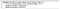Source (Dense block)

The causal attention layer in SNAIL is responsible for creating self-attention similar to the Transformer used in BERT.Attention in the Transformer

The general principle allows the model to focus (pay attention) on subparts of the input features.

Queries q, keys k, and values v are generated (learned) from the input using different learned affine transformation (linear transformation).

The queries symbolize what we are interested in and the keys encode the values v information. We multiply q and k together to estimate where the needed focuses to be. And then, we mask out the values v that we should not pay attention to.

This is an extremely rough explanation of self-attention, please refer to the attention in this article for details. And here is the pseudocode in performing the self-attention.

For casualty, CausallyMaskedSoftmax(·) zeros out the appropriate probabilities before normalization such that the query cannot have access to future keys/values.

Recap

Here is the major difference between the optimizer approach and the model-based approach. In the model-based approach, the meta-learner predicts/adapts the model parameters for the learner using samples from the support, i.e. 𝜙ᵢ f(support samples) with f parameterized by θ. In optimizer, we use methods like gradient descent to refine θ to become 𝜙ᵢ with the support samples.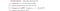Source

For the LSTM-based Meta-Learner, it uses a DNN to adjust θ (with inputs including the loss gradient) instead of performing gradient descent directly.

But this DNN f approach may encounter one issue. If the DNN has not been explored with tasks similar to Dtr_i, there is no promise of the accuracy of its predictions. As shown below, when the input character is smeared further, the accuracy drops for SNAIL and MetaNet (both using recurrent based DNN). This is because the input is out-of-distribution from how the DNN was trained. It shows less generalization compared with a gradient-based optimizer. On the contrary, MAML has a better inductive bias that can generalize and extrapolate to unforeseen tasks better.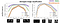Source

A consistent meta-learner will converge to a local optimal on any new tasks, regardless of the meta-learner model. An gradient descent based optimizer solution is a consistent meta-learner as it uses gradient descent to improve the model. Even it gets a bad start from the meta-learning, it can still converge to at least a local optimal.

But for model-based models, it is not consistent. If it has not been exploited and explored properly near the data space of the new tasks, we will not reach the local optimum.

Reptile (paper)

In MAML, we apply a derivative in the inner loop for each task and another derivative for the meta-learner, so it is a second-order derivative. Unfortunately, the second-order derivate may exhibit instabilities in training. FOMAML (First order MAML) simplifies the gradient calculation by simplifying the first gradient term below (w.r.t. model parameters) to contain all one.

Therefore, the gradient of FOMAML will be calculated from the second term only — a first-order loss function derivative using the updated model and testing sample B. This simplification will work well with many meta-learning problems with the exception of reinforcement learning and imitation learning. Other approaches in addressing the instability problem may involve different learning rates or training strategies between the inner loop and the outer loop.

In Reptile, this gradient calculation is even further simplified. Reptile performs a k-step model update and uses the difference in the last model and the original model as the gradient in the gradient descent.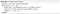Source

Consider the optimal for task 1 and task 2 lay on the surface of W₁ and W₂ respectively. So Reptile moves 𝜙 towards the area that is closest to those boundaries.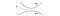Source

Meta-Learning Priors

MAML is model agnostic. Without further proof here, MAML with gradient descent and early stoping imply a Gaussian prior for p(𝜙ᵢ | θ) with means around θ.

This finding implies the possibility that we can implicitly define the type of prior and the corresponding learner’s ML model. For example, ALPaCA learns a feature encoding, as well as a prior p(W) generated from a meta-learner’s NN. For example, this NN output the mean and variance of a Gaussian for each weight to be used in the Bayesian linear regression. So, once features are extracted, ALPaCA computes the posterior (the prediction distribution) by applying Bayesian linear regression with the prior. For your reference, below is a general description of the Bayesian linear regression.Bayesian linear regression algorithm

By splitting the process into feature extraction followed by a Bayesian linear regression, it makes the calculation to be tractable.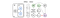Source

To demonstrate the idea of creating a meta-learner to generate model parameters for a specific optimization method (say Bayesian linear regression or SVM), we will detail the MetaOptNet. We pick this model because it is start-of-the-art technology in 2019 on meta-learning datasets and benchmarks.

MetaOptNet (paper)

MetaOptNet extracts feature from the input image and for the last layer, it learns a Base learner A to estimate the SVM weights (instead of Bayesian regression’s parameters in ALPaCA). These weights will multiply with the embedded features in the query (bitwise) in making classification predictions. This linear predictor, implemented as SVM, processes a nice generalization that results in state-of-the-art performance.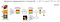Modified from source

The key objective in MetaOptNet is to learn feature embeddings that generalize well under a linear classifier (SVM). A linear base learner for classification is selected because the objective function for such a learner is convex and differentiable. How to optimize this function is not only studied heavily but can be optimized efficiently.

Below are the objectives for the base learner and the meta-learner. It just looks complicated but it is pretty simple. It uses the SVM penalty (the hinge loss) to train the base learner.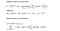Source (Please refer to the source for the term definitions. K is the number of classes in the support)

The meta-learner objective is just a re-expression of

which finds the best feature extractor to work with the SVM and the testing data.

Self-Critique and Adapt (SCA)

As discussed before, in the inner loop of many meta-learning algorithms, we use N-step gradient descent with the support samples to improve a learner θᵢ (step ① below).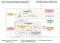Source

In SCA, it also learns a critic C parameterized by W to judge how good θᵢ is. The input to C is F. F summarizes (or extract) the model θᵢ (step ②) and may include other parameters like the predictions on the query and the embedding context of the support. After computing the score in Critic C (step ③), its gradeint will be used to improve θᵢ with gradient descent. Step ② to step ④ will be repeated for I times to improve the model. In short, the critic result is used to improve the one being criticized. Critic C is modeled without the true labels as input and therefore, it is called the label-free critic model. So, how can we train critic C if the true lables are not visible from Step ③ to step ④.

The short answer is we just delay the complete training to a later step. Once the improvement on θᵢ is commenced. SCA computes the loss on the model θᵢ’s predictions using the query true labels. The corresponding gradient will be backpropagated to train all the NNs involved in the process (step ⑤). This will include the critic model.

In meta-testing, we will use the critic C to improve θᵢ after adapting it with support samples in the meta-testing.

# Metric Learning/Non-parametric Model

What is the difference between a parametric model and a non-parametric model? Parametric algorithms learn models to capture knowledge. Once a model is built, we make predictions using its parameters and we can throw away the training data. On the other hand, non-parametric models keep the data. To make predictions, we explore the similarity of the input with the accumulated samples. One of the most well-known non-parametric models is the KNN (K Nearest Neighbors) which uses the labels of the closest neighbors in making predictions. Non-parametric models may have problems with huge datasets. But it works well with Meta-Learning as the support usually contains very few samples.

In Metric Learning, the knowledge in the meta-training dataset is still captured by a parametric model, which is often in the form of feature extractors. But to adapt to a specific task, we explore similarities for the query with the labeled support. In this process, there are two questions that need to be answered: what are we comparing and how are we comparing. If we compare images pixel by pixel with L2 distances, we are going to fail.

Siamese Neural Networks (paper)

One of the most critical tasks in DL is feature extraction. If we want to generalize a classifier, the feature extractor must capture general knowledge that distinguishes classes. In a Siamese Neural Network, it uses two identical networks, sharing the same model parameters, to extract features for two samples. Then we feed the extracted features into a discriminator to tell whether both samples belong to the same class or not.

In the original paper, L1 distancing is used to measure the distance between two feature vectors. Then it is feed into a classifier (say a fully-connected layer) in determining whether they belong to the same class. But other distance metrics like the cosine similarity or L2 can be used. If the objects belong to the same class, output p should be close to 1, otherwise 0. Therefore, by computing a loss function based on the true labels and the predictions on different tasks, we train the feature extractor to extract basic features that distinguish general objects.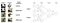Modified from source

The diagram below shows an example of using a CNN network to extract features from an image.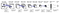Source

Matching Network (paper)

Matching Network compares an image from the query with each image in the support. Similarities are measured with the cosine similarity after the images are encoded by f and g respectively. Then the values are normalized by the softmax function into a probability. In each task, we apply one-shot learning to map a query object to one of the classes in the support (say, German Sheperd).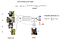Source

The model is trained to maximize the probability of the true label while minimizing others. f and g are the feature extractor that we train. One popular realization is to have both f and g share the same parameters and implemented them as a CNN.

Fully Conditional Embedding

However, both f and g encode samples independently of others and without the context of the support S. This may hurt if images in the support are in similar subcategories, like different breeds of dogs, with many similarities. In this situation, the encoding should be sensitive to the support and extract features that can distinguish them. This requirement will be addressed by the encoding method called Fully Conditional Embedding.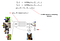Modified from source

In ① above, we apply g’(xᵢ) to extract image features from xᵢ — image i in the support. Usually, this is done with a CNN network. Then the features are feed into a bi-directional LSTM.

The coding g for xᵢ will be g(xᵢ, S) instead of g(xᵢ), i.e. the encoding of xᵢ will be sensitive to the support. The encoding g(xᵢ, S) is the addition of g’(xᵢ) with the hidden states hᵢ of LSTM cell i in both forward and backward direction. Since cells state cᵢ contains knowledge for the images processed so far, the encoding in hᵢ will be trained to encode data with the account of the support.

The Fully Conditional Embedding f encodes a query image using attention with LSTM.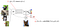Modified from source

f’ extracts the image features from the query image. Again, this can be done with a CNN network. Then we apply a K step “read” using an LSTM on f’, and the context of the support. The key idea is to provide an encoding scheme that pays attention to images in the support such that the scheme can be self-adjusted to handle the subtlety among the support images.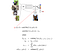Modified from source

In specific, the hidden state in each LSTM cell is sensitive to the readout r in the last time step. This readout is based on the similarity between its hidden state and g(xᵢ) where xᵢ is the image i in the support. This encoding repeat for K timesteps and the final hidden state is the embedding f for the query image. The equations are a little bit entangled but the general principle is pretty simple. At every timestep, it pays more attention to similar images in the support and moves the extracted features closer to these features. It is repeated K times to refine the process. So the extracted features for the query will be features that close to its similar images in the support.

The details of this K step “Process” block can be found here and this is the general equations.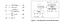Source

Relation Network (paper)

In Relation Network, the encoding of each image in the support is concatenated with the query’s encoded features. Then the DNN g is used to score their similarity. Then, it uses the highest score to associate a query to an image in the support.

Prototypical Networks (paper)

The algorithm in Prototypical Networks is very similar to clustering. The key idea is to find the centroid for objects belonging to the same class. We train an embedding function f to encode images. The centroid of objects belonging to the same class is computed by averaging their encoded features. A prediction is made by a softmax function measuring the inverse of the distance from each centroid.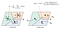Source

# Model-based v.s. optimization-based v.s. non-parametric model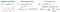Source

Let’s compare all three meta-learning approaches a little bit. The model-based method can be modified in solving other AI domains, like reinforcement learning. But the training often starts from scratch without any hints on where to look first, i.e. no inductive bias, at least in the beginning. Therefore, it is sample inefficient. The optimization-based model can handle varying and large K well (K-shot) and it can make reasonable good extrapolation on out-of-distribution tasks. But it may have stability problems because of the 2nd-order optimization. But this issue can be mitigated. Non-parametric models are computationally fast and simple but it is harder in handling varying or large K, as shown in the empirical results. And it is mainly for classification only.

# Next

Next, we will cover Bayesian Meta-learning, Unsupervised, and Weak supervised Meta-Learning. Bayesian allows the algorithms to handle uncertainty in real life and weak supervised learning addresses the expensive cost of collecting samples.

# Credits and References

Meta-Learning: from Few-Shot Learning to Rapid Reinforcement Learning

Deep Learning